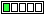All about flooble | fun stuff | Get a free chatterbox | Free JavaScript | Avatarsperplexus dot infod1 or more ? (Posted on 2016-03-07)A problem from the 2004 Harvard-MIT Math Tournament:

Zach chooses five numbers from the set {1, 2, 3, 4, 5, 6, 7} and tells their product to Claudia. She finds that this is not enough information to tell whether the sum of Zach’s numbers is even or odd. What is the product that Zach tells Claudia?

I believe it is a d1 puzzle.

Are you on my side?

 See The Solution Submitted by Ady TZIDON No RatingComments: ( Back to comment list | You must be logged in to post comments.)My Analysis| Comment 2 of 4 |Each product of five of the numbers corresponds one-to-one to the products of the two numbers left out.  Specifically, (Product of 5 chosen) * (Product of two left out) = 7! = 5040.

If Claudia cannot tell the parity of the sum of the five numbers then she also cannot tell the parity of the sum of the two numbers left out.  The parity of those two sums must be the same since the sum of both sets is 1+2+3+4+5+6+7 = 28.

There are only two products that can be made two different ways from the set {1,2,3,4,5,6,7}.  They are 6 = 1*6 = 2*3 and 12 = 2*6 = 3*4.  Both sums of factors of 6 have odd parity, so is not the answer.  This leaves 12, whose sums of factors are of opposing parity.

Therefore Zach told Claudia the number 5040/12 = 420.

I would rate this puzzle as a D2.  If the puzzle stated that Zach gave Claudia a two term product then I would rate it D1.  But with a 5 term product I would expect a solution that either takes the extra step converting the puzzle to the 2 term product like I did, or go through a more direct effort sifting through all the 5 term products directly which very likely makes it need at least a paper-and-pencil approach to deal with the larger numbers as opposed to easy mental math with 2 term products.

 Posted by Brian Smith on 2016-03-07 10:20:44Please log in:

 Search: Search body:
Forums (0)